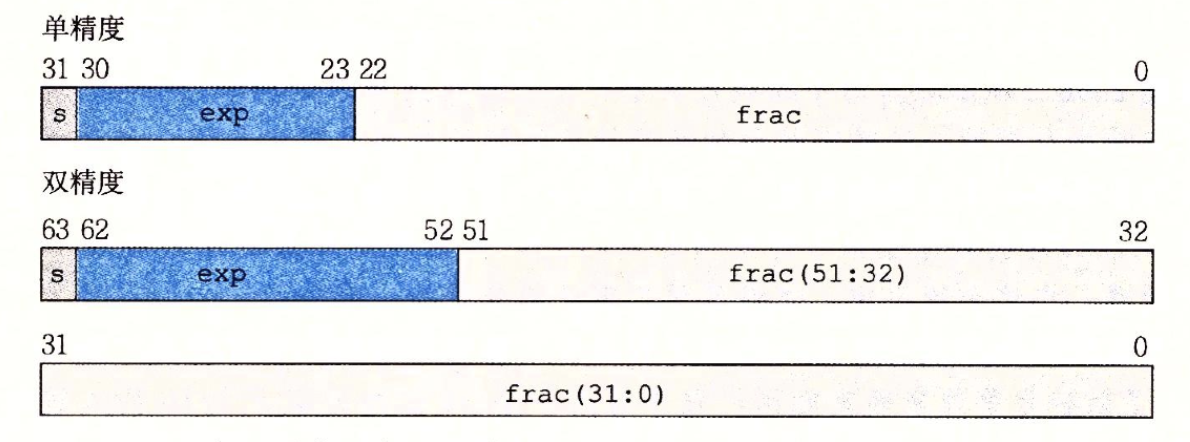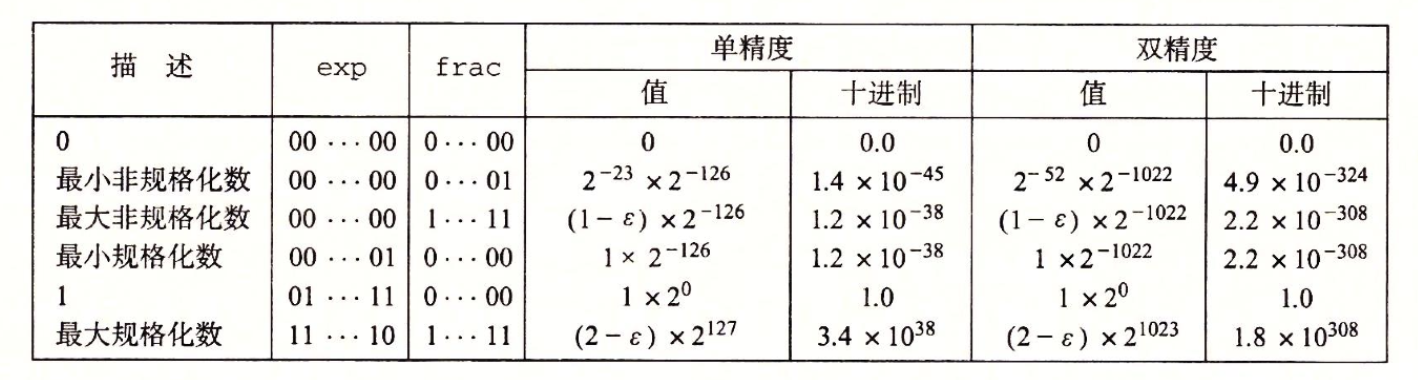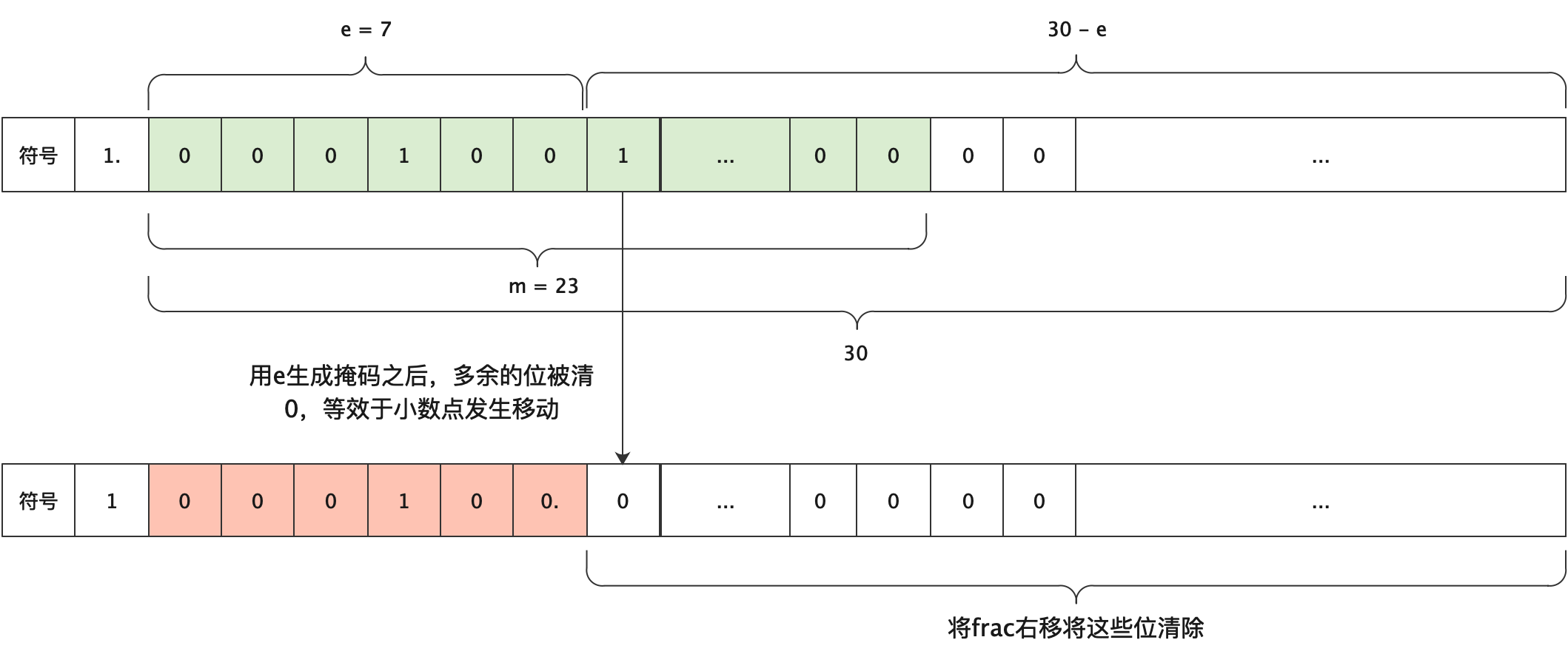# Justin Lei

Study forever

## 阅读笔记

### 布尔运算及定律

• 吸收律：$A+(A * B) = A$，$A * (A + B) = A$

• 分配律：$A(B+C) = AB + AC$，$A+(B*C) = (A+B)(A+C)$

• 德摩根定律：$\overline{AB} = \bar{A}+\bar{B}$​​​，$\overline{A+B} = \bar{A}\bar{B}$

### 异或

a ^ b

= ~a · b + a · ~b

= a * ~a + b * ~b + ~a * b + a * ~b = (a + b) * (~a + ~b)

= (a + b) * (~(a * b))

// 设origa代表a的初始值，origb代表b的初始值
b = a ^ b; // 此时b = origa ^ origb
a = a ^ b; // 此时a = origa ^ origa ^ origb = 0 ^ origb = origb
b = a ^ b; // 此时b = origb ^ origa ^ origb，由于


## 常见位运算

• 对于有符号数来说，~x = -(x + 1)

• 自增1

允许负号：-~num，不允许：num + 1

• 自减1

允许负号：~-num，不允许：~(~num + 1)

• 判断x == y

!(x ^ y)

• 判断一个数是否小于0

!(x >> 31)

• 找到最高为1的位对应的阶数

// 32bits
n |= (n >> 1);
n |= (n >> 2);
n |= (n >> 4);
n |= (n >> 8);
n |= (n >> 16);
return n - (n >> 1);


本质上是利用二分法将MSB为1的位向右传播，最终n会变为000111111…1的形式，然后计算出1对应的阶数

• 找到最低位为1对应的阶数

n & (n - 1)

• 二分法查找最高为1的位的索引

// 输出索引
uint highest_one_idx(uint x) {
if (x & 0xffff0000U) { x >>= 16; r += 16; }
if (x & 0x0000ff00U) { x >>= 8;  r += 8; }
if (x & 0x000000f0U) { x >>= 4;  r += 4; }
if (x & 0x0000000cU) { x >>= 2;  r += 2; }
if (x & 0x02)        {					 r += 1; }
return r;
}

// 不能使用if
uint highest_one_idx(uint x) {
int tmp = 0, idx = 0;
// 等价于if (x > 0xffffu)
tmp = (~((x >> 16) + ~0) >> 27) & 0x10;
idx += tmp, abs >>= tmp;
// if (x > 0xffu)
tmp = (~((x >> 8) + ~0) >> 28) & 0x8;
idx += tmp, abs >>= tmp;
// if (x > 0xfu)
tmp = (~((x >> 4) + ~0) >> 29) & 0x4;
idx += tmp, abs >>= tmp;
// if (x > 0x3u)
tmp = (~((x >> 2) + ~0) >> 30) & 0x2;
idx += tmp, abs >>= tmp;

// if (x == 1)
idx += (x & 0x02) >> 1;

return r;
}

• 二分法找到$log_2^n$

unsigned int ulog2 (unsigned int u)
{
unsigned int s, t;

t = (u > 0xffff) << 4; u >>= t;
s = (u > 0xff  ) << 3; u >>= s, t |= s;
s = (u > 0xf   ) << 2; u >>= s, t |= s;
s = (u > 0x3   ) << 1; u >>= s, t |= s;

return (t | (u >> 1));
}

// 替换掉>号，和highest_one_idx相同
unsigned int ulog2 (unsigned int u)
{
unsigned int r = 0, t;

t = ((~((u >> 16) + ~0U)) >> 27) & 0x10;
r |= t, u >>= t;
t = ((~((u >>  8) + ~0U)) >> 28) &  0x8;
r |= t, u >>= t;
t = ((~((u >>  4) + ~0U)) >> 29) &  0x4;
r |= t, u >>= t;
t = ((~((u >>  2) + ~0U)) >> 30) &  0x2;
r |= t, u >>= t;

return (r | (u >> 1));
}


### 位运算推荐资料

1. 《Hacker’s Delight》这本书里面介绍了很多高效的算法

2. 《Matters Computational》有一章专门介绍各种bitwise算法

3. https://graphics.stanford.edu/~seander/bithacks.html

### 浮点数

IEEE的浮点数表示，浮点数格式为$(-1)^s \times M \times 2^E$，其中s表示符号位，M由$f_{n-1}f_{n-2}…f_0$​​组成，为二进制小数，被称为尾数，E被称为阶数，由exp表示。1. 规格化：0 < exp < $2^k-1$，此时实际的阶数$E=exp - bias$​​，$bias = 2^{k-1} - 1$​​，单精度中为127，双精度中为1023；M表示$1.f_{n-1}f_{n-2}…f_0$​​，即隐含了个位数的1。
2. 非规格化：exp == 0，此时阶数$E = 1-bias$​，M表示$0.f_{n-1}f_{n-2}…f_0$​​，此种格式用于表示0<num<1的浮点数。
3. exp全为1，此时如果frac为0，表示+∞或者-∞，如果不为1，则表示NaN，即数据出错（not a number），可以用来表示非法计算结果或者未初始化结果。

#### 浮点数的极值## DataLab

### isTmax

!(~(x + !(x + 1)) ^ (x + 1))


### isLessOrEqual

less分为两种情况，一种是符号位相同，只需比较x-y < 0，另一种是符号位不同，比较符号位即可。

equal使用!(x^y)判断。为了减少操作数，取符号数的移位操作可以放到最后再执行

  int res = x ^ y;
int flag1 = (~res) & (x + ~y + 1); // 符号相同，判断x-y < 0
int flag2 = x & (~y); // 符号不同，判断符号
int equal = !res;
return equal | ((flag1 | flag2) >> 31 & 1);


### logicalNeg

  int sign = (x >> 31) & 1; // 判断是否是负数
int nonzero = ((x ^ (~x + 1)) >> 31) & 1; // 判断是否为0
return (~(sign | nonzero)) & 1; // 判断符号位


  int res = x ^ (~x + 1);
return (~(res | x) >> 31) & 1;


### howManyBits

1. 如果x是正数，则需要多一个符号位

2. 如果x是0，则不需要符号位

3. 如果x是负数，当求出来的索引对应的负数大于x本身时，需要向前再多一位，也即

-(1 « idx) > x，等价于(x + (1 « idx)) < 0

4. 如果x是Tmin，由于int的范围是Tmin <= x <= -Tmin + 1，因此绝对值会超出范围，所以需要单独讨论，如果是Tmin就返回32

int howManyBits(int x) {
int mask = (x >> 31);
int positive = ((!!x) & !(mask & 1));
int idx = 0, tmp = 0;
int isMin = !(x ^ (1 << 31));

// 计算最高位的索引
tmp = (~((abs >> 16) + ~0) >> 27) & 0x10;
idx += tmp, abs >>= tmp;
tmp = (~((abs >> 8) + ~0) >> 28) & 0x8;
idx += tmp, abs >>= tmp;
tmp = (~((abs >> 4) + ~0) >> 29) & 0x4;
idx += tmp, abs >>= tmp;
tmp = (~((abs >> 2) + ~0) >> 30) & 0x2;
idx += tmp, abs >>= tmp;
idx += (abs & 0x02) >> 1;

isMin = ~isMin + 1;

return (isMin & 32) + (~isMin & (idx + (positive | ((x + (1 << idx)) >> 31 & 1)) + 1));
}


### floatScale2

unsigned floatScale2(unsigned uf) {
unsigned e = (uf >> 23) & 0xffu;
unsigned mmask = (1 << 23) - 1;
unsigned m = uf & mmask;
unsigned newe = 0, newm = m;
unsigned scale2m = 2 * m, beyond = (scale2m >> 23) & 1;
unsigned inm = scale2m & mmask;
if (e == 0xffu) return uf;
// 非规格化，需要判断乘于2是否满足规格化
if (e == 0) {
newm = inm;
if (beyond) newe = 1;
} else {
// 规格化，直接递增e
newe = e + 1;
if (newe == 0xffu) {
newm = 0;
};
}
return (uf & (1 << 31)) | (newe << 23) | newm;
}


### floatFloat2Intint floatFloat2Int(unsigned uf) {
unsigned e = (uf >> 23) & 0xffu;
unsigned frac = (uf & ((1 << 23) - 1));
unsigned sign = (uf >> 31) & 1;
// 判断inf/nan
if (e == 0xffu) return (1 << 31);
// 非规格化
if (e == 0) return 0;
// 规格化，加上隐含的1，扩充到31位方便后续处理
frac = (frac | (1 << 23)) << 7;
if (e < 127) return 0; // 小数返回0
e -= 127;
// e可能非常大，需要判断是否超出范围，由于预留符号位，所以e不可能超过30
if (e > 30) return 1 << 31;
mask = (-1 << (30 - e));
num = ((frac & mask) >> (30 - e));
if (sign) num = ~num + 1;
return num;
}


### floatPower2

unsigned floatPower2(int x) {
unsigned e = 0, frac = 0;
// 非规格数最小到2^-149
if (x < -149) return 0;
// 规格数最大到2^127
if (x > 127) return 0x7F800000u;

if (x >= -126) {
e = x + 127;
} else if (x < -126) {
frac = (1 << (149 + x));
}
return (e << 23) | frac;
}